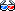This version (2017/06/04 23:35) was approved by zdimension.The Previously approved version (2017/06/04 19:00) is available.# World Layout

## Coordinate System

The engine uses a right-handed coordinate system, with $X$ to the right, $Y$ down, and $Z$ forward.

The mapping between OpenGL and the engine's coordinate system is $(x, y, z)_\mathrm{gl} = (x, -y, -z)$.

### Units

The most important “metering” in the engine is the sector size, which defines a length of $1024$ units, and approximately equals two meters in the real world.

Another significant, but less important, metering is the click, which is a quarter sector size ($256$ units). It is used to define floor and ceiling slants, and is also a “rule of thumb”

### Sectors

The whole engine world is divided into sectors. A sector (which defines a $1024 \times 1024$ rectangular area) defines

• ceiling and floor layout,
• behaviour, and
• the adjoining room for that sector.

## Angles“AU” is short for “Angular Unit”. $1$ AU equals the $2^{16}$th of a full circle and ranges from $-2^{15}$ ($-32768$) to $2^{15}-1$ ($32767$), representing the interval $[-180^°; 180^°[$.

The conversion functions are:
$\varphi_{\mathrm{rad}} = \varphi_{\mathrm{deg}} \cdot \pi / 180 = \varphi_{\mathrm{AU}} \cdot \pi / 2^{15}$
$\varphi_{\mathrm{deg}} = \varphi_{\mathrm{rad}} \cdot 180 / \pi = \varphi_{\mathrm{AU}} \cdot 180 / 2^{15}$
$\varphi_{\mathrm{AU}} = \varphi_{\mathrm{deg}} \cdot 2^{15} / 180 = \varphi_{\mathrm{rad}} \cdot 2^{15} / \pi$

### YPR Rotation

The engine uses Euler angles to express rotations, and applies them in ZXY order.

## Rooms & Portals

The world is divided into so-called rooms, which are basically subdivisions of the whole level. A room may be physically incomplete, i.e. a large physical room may be artificially split into two engine rooms. Each room is connected to its direct neighbors through portals. The engine uses these visibility portals to determine which room should be rendered and which not.

See https://en.wikipedia.org/wiki/Portal_rendering for a more in-depth explanation.